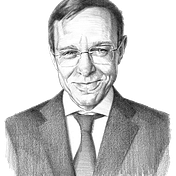# The Most Fantastic Spacecraft Ever Imagined

After a snow storm, there is no greater thrill than collecting fluffy snow to make a snowball and throw it away at a high speed. Similarly, in an accelerating universe — there is no greater thrill than collecting dark energy to make an object of negative mass that launches a spacecraft to the speed of light. You must be thinking: “Wait, what?” Let me explain.

Legend has it that Isaac Newton formulated the theory of gravity after watching an apple fall off a tree and asking why the apple fell straight down. Newton reasoned that the force of gravity attracts the apple to the Earth, as it attracts to each other any two objects of positive mass. Albert Einstein generalized Newton’s theory by interpreting gravity as the curvature of spacetime, to which all test objects respond in the same way. This stems from the so-called Equivalence Principle, by which the inertial mass of an object, dictating its acceleration in response to a force acting on it, equals its gravitational mass, namely its coupling to gravity.

But Einstein’s reformulation of gravity also led to a surprise. Einstein’s equations predict that under the dominance of a vacuum energy density, like the dark energy observed in the present-day Universe, an apple would accelerate away from Newton. Hence, gravity can be repulsive. Distant galaxies are observed to accelerate away from us for this reason. We still do not understand the nature of dark energy. To explain it, we might need to develop a predictive theory of quantum gravity.

Even though physicists were not successful in unifying quantum mechanics and gravity over the past century, we should remain optimistic about the future prospects of physics. Suppose that millennia from now, quantum-gravity engineers will build a dark-energy plow that manufactures objects of negative mass out of the natural cosmic reservoir of dark energy surrounding us. What would that imply?

A negative-mass object would accelerate opposite to the direction of an external force. If we push it away, it will accelerate towards us. According to the equivalence principle, the negative-mass object will also couple to gravity with an opposite sign, namely it will repel positive-mass objects.

Now, imagine placing the negative-mass object next to an equal but opposite positive-mass object. The gravitational repulsion between the masses will push the positive mass away but will also pull the negative mass to chase it in the same direction. As a result, the two masses will move at a constant acceleration along the direction of the axis that connects them. The pair will accelerate together to the speed of light in a runaway process. The pair’s total mass is zero, so there is no need for fuel.

Case closed. Wouldn’t you agree that there is no bigger thrill than creating an ideal spacecraft out of a pair of equal but opposite masses? This fantastic concept for a spacecraft was first conceived in a 1957 paper by Hermann Bondi and further studied by Robert Forward in a 1990 paper.

Obviously, there are conceptual and practical problems with this “free-lunch” concept for propulsion. First, a pair of equal but opposite masses costs no energy because it has a zero total mass. Hence, the vacuum could spontaneously produce pairs of positive and negative masses. The pairs would accelerate to the speed of light and fill up the Universe. The fact that we do not observe them means that either negative masses are forbidden in nature or there is a quantum-number barrier to their spontaneous creation. On the practical level, a spacecraft with an exactly zero net mass would decelerate considerably as a result of a collision with a single gas or dust particle in the interstellar medium. This is in contrast to a rocket which allows for a steady acceleration of a finite positive mass.

In particular, if a spacecraft accelerates at the terrestrial gravitational acceleration of 9.8 meters per second squared, then an astronaut on board would have the same experience as being on the surface of Earth. Maintaining this constant acceleration over several decades will bring the astronaut sufficiently close to the speed of light, so as to maintain enough time dilation and traverse the entire Universe during a human’s lifetime.